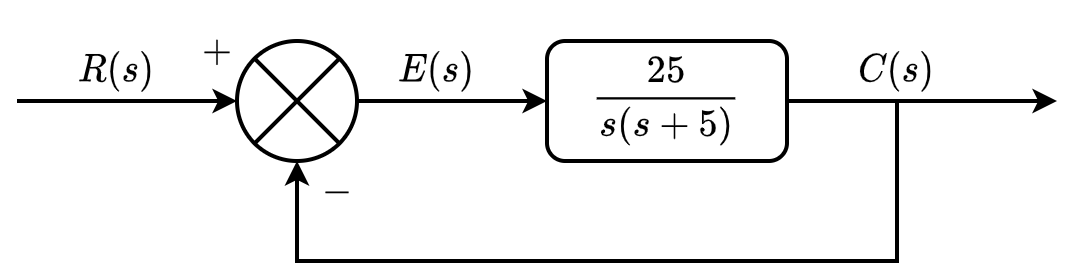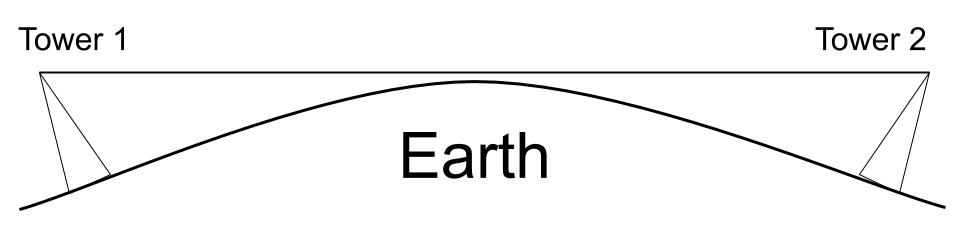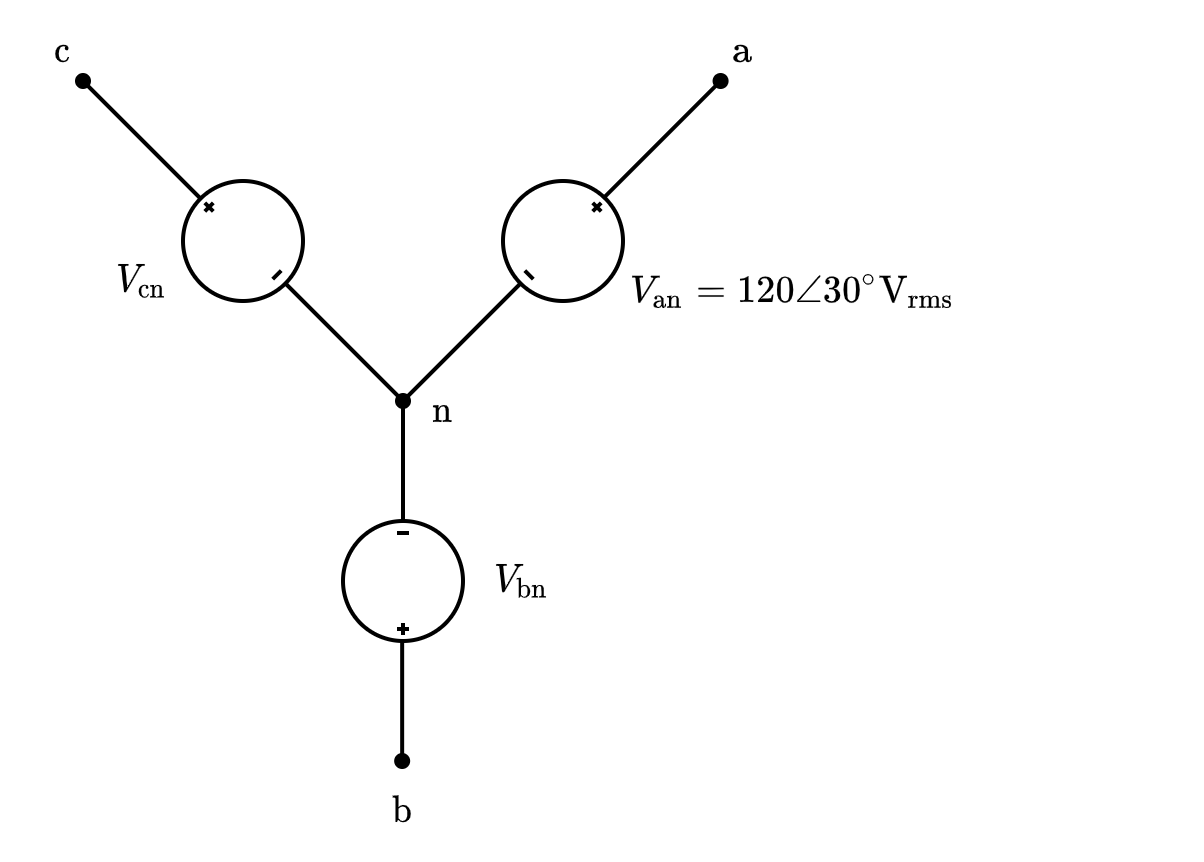Free FE Practice Test

# PrepFE™

## Free FE Electrical and Computer Example Practice Problems

We've selected 10 diverse practice problems from our question bank that you can use to review for the Electrical and Computer engineering FE exam and give you an idea about some of the content we provide.

### 1) Obtain the homogenous solution to the differential equation that yields the voltage, $v_C(t)$ across the capacitor in the series RLC circuit. $i_L(0) = 0.25 \si A$ and $v_C(0) = 0$.### 3) In the circuit shown, find the power absorbed by the dependent source.### 5) For the given circuit, $R_1 = R_3 = 5 \si {k\Omega}$. Determine $R_2$ and $R_4$ to obtain a gain of $10$.### 6) For the system shown in the figure, compute the peak value time:## Solutions

### 1) Obtain the homogenous solution to the differential equation that yields the voltage, $v_C(t)$ across the capacitor in the series RLC circuit. $i_L(0) = 0.25 \si A$ and $v_C(0) = 0$.A.$e^{-\frac{1}{2}t} \text{ V}$
B.$e^{-\frac{1}{2}t}\sin\frac{1}{2}t + \cos\frac{1}{2}t \text{ V}$
C.$\sin\frac{1}{2}t \text{ V}$
D.$e^{-\frac{1}{2}t}\sin\frac{1}{2}t \text { V}$

### Explanation:

Refer to the Differential Equations section and the Second-Order Linear Homogeneous Differential Equations with Constant Coefficients section in the Mathematics chapter of the FE Reference Handbook.

The homogenous solution is to be obtained. The homogenous solution is when the input function, the voltage source $v_s(t)$ in this case, is set equal to $0$. Therefore, we can write the loop equation for the RLC series circuit as, $$v_L + v_R + v_C = 0$$ Express $v_L$ as $v_L = L\frac{di_L}{dt}$ (the voltage across an inductor equation), and express $v_R$ as $v_R = i_LR$ (Ohm's Law).

Now, substitute $C\frac{dv_C}{dt}$ for $i_L$ (the current through the capacitor is the same as the current through the inductor since the elements are in series) to obtain the full homogenous equation in terms of $v_C$, $$LC\frac{d^2v_C}{dt^2}+RC\frac{dv_C}{dt} + v_C = 0$$ Divide through by $LC$, $$\frac{d^2v_C}{dt^2}+\frac{R}{L}\frac{dv_C}{dt} + \frac{1}{LC}v_C = 0$$ This is now a second order linear homogenous differential equation with constant coefficients in the form, $$r^2 + ar + b = 0$$ To determine the form of the solution for this second order linear homogenous equation with constant coefficients, compute if $a^2 \gt 4b$, or if $a^2 = 4b$, or if $a^2 \lt 4b$.

In our circuit, $a$ = $\frac{R}{L}$ = $\frac{(4\Omega)}{(4 \si H)} = 1$, and $b$ = $\frac{1}{LC} =\frac{1}{(4 \si H)(0.5 \si F)} = \frac{1}{2}$

Now determine $a^2$ and $4b$ and compare. $$a^2 = 1^2 =1$$ $$4b = 4(\frac{1}{2}) = 2$$ Because $a^2 \lt 4b$, the solution will take the form, $$y= e^{\alpha x} (C_1 \cos \beta x + C_2 \sin \beta x)$$ Where $\alpha$= – $\frac{a}{2}$ and $\beta$ = $\frac{\sqrt{4b-a^2}}{2}$

Compute $\alpha$, $$\alpha = -\frac{a}{2} = -\frac{1}{2}$$ Compute $\beta$, $$\beta = \frac{\sqrt{4b-a^2}}{2} = \frac{\sqrt{4(0.5)-1^2}}{2} = \frac{1}{2}$$ Thus the expression for $v_C(t)$ becomes, $$v_C(t) = e^{-\frac{1}{2}t}\left(C_1\cos\frac{1}{2}t+C_2\sin\frac{1}{2}t\right)$$ When $t = 0$, $v_C(0) = 0 = C_1$, then use $i_L$ to find $C_2$ as follows, $$\frac{i_L}{C} = \frac{dv_C}{dt} = -\frac{1}{2}e^{-\frac{1}{2}t}C_1\cos\frac{1}{2}t \\ - \frac{1}{2}e^{-\frac{1}{2}t}C_1\sin\frac{1}{2}t - \frac{1}{2}e^{-\frac{1}{2}t}C_2\sin\frac{1}{2}t+\frac{1}{2}e^{-\frac{1}{2}t}C_2\cos\frac{1}{2}t$$ Substitute the initial condition at $i_L(0)$, $$\frac{i_L(0)}{C} = \frac{dv_C(0)}{dt} = -\frac{1}{2}C_1 + \frac{1}{2}C_2$$ $$\frac{i_L(0)}{C} = \frac{0.25\si A}{0.5\si F} = \frac{1}{2} = -\frac{1}{2}C_1 + \frac{1}{2}C_2$$ Since $C_1$ is known to be $0$, then $C_2 = 1$.

Finally, substituting the values for $C_1$ and $C_2$ into the expression for $v_C(t)$ we found earlier yields the homogeneous solution, $$v_C(t) = e^{-\frac{1}{2}t}\sin\frac{1}{2}t \text{ V}$$

### 2) A magnetic field has the vector field $\mathbf{\vec B} = 10y \mathbf i +2y \mathbf j - mz \mathbf k$. The constant "m" is most nearly:

A.$-2$
B.$4$
C.$0$
D.$2$

### Explanation:

Refer to the Electromagnetic Dynamic Fields section in the Electrical and Computer Engineering chapter of the FE Reference Handbook.

The divergence of a magnetic field is defined to be 0 by Maxwell's Equations. $$\nabla \bullet \mathbf B = 0$$ Therefore, calculate the divergence and set the expression equal to zero.

The divergence of a vector field is defined as, $$\nabla\bullet \mathbf V = \left (\frac {\partial}{\partial x}\mathbf i\ +\frac {\partial}{\partial y}\mathbf k +\frac {\partial}{\partial z}\mathbf j \right) \bullet (V_1 \mathbf i + V_2\mathbf j + V_3\mathbf k)$$ Thus, $$\nabla \bullet \mathbf B = \frac{d}{dx} 10y \mathbf i + \frac{d}{dy} 2y \mathbf j - \frac{d}{dz} mz \mathbf k = 0$$ $$\nabla \bullet \mathbf B = 0 + 2 - m = 0$$ $$m=2$$

### 3) In the circuit shown, find the power absorbed by the dependent source.A.$-67.7\si{W}$
B.$67.7\si{W}$
C.$1690\si{W}$
D.$-1690\si{W}$

### Explanation:

Refer to the Power Absorbed by a Resistive Element & Kirchhoff's Laws sections in the Electrical and Computer Engineering chapter of the FE Reference Handbook.

Begin by writing a KVL (loop) equation for the single loop. Assume the current flows in the clockwise direction. Note that it will ultimately not matter which direction the current is assumed to flow as shall be explained at the bottom. This yields, $$150\si{V} +0.8v_x - 20\si{V} = i(10\Omega + 50\Omega)$$ Note that the $v_x$ voltage is equal to $(i)(50)\Omega$ (by Ohm's law, the current flowing in the resistor is $i$ and the resistor voltage, $v_x$, is defined as the voltage drop across the $50\Omega$ resistor) so it may be substituted directly into the loop equation to yield, $$150\si{V} + 0.8(50i) - 20\si{V} = i(10\Omega + 50\Omega)$$ Solving for the unknown, $i$, produces, $$150\si{V} - 20\si{V} = i60\Omega - i40\Omega$$ $$130\si{V} = i20\Omega$$ $$i = \frac{130\si{V}}{20\Omega} = 6.5\si{A}$$ For a source to absorb power, then positive current must flow out of the negative terminal. For the dependent source, current flows out of the positive terminal. If the current had been assumed to be counterclockwise, then the current would have been negative and a simple sign reversal would yield the same result as below.

Thus, $$P = IV = -(0.8v_x)(6.5\si{A})$$ We know that $v_x = 50\Omega i = (50\Omega)(6.5 \si A)$. Therefore, $$P = -[(0.8)(50\Omega)(6.5\si{A})(6.5\si{A})] \\ = -1690\si{W}$$

### 4) Pipelining improves CPU performance by enabling the processor to:

A.reduce memory access time.
B.perform parallel processing.
C.increase clock speed.
D.increase the number of functional units.

### Explanation:

Pipelining is an implementation technique where multiple instructions are overlapped in execution. The computer pipeline is divided in stages. Each stage completes a part of an instruction in parallel. The stages are connected one to the next to form a pipe - instructions enter at one end, progress through the stages, and exit at the other end. Pipelining does not decrease the time for individual instruction execution. Instead, it increases instruction throughput. The throughput of the instruction pipeline is determined by how often an instruction exits the pipeline.

### 5) For the given circuit, $R_1 = R_3 = 5 \si {k\Omega}$. Determine $R_2$ and $R_4$ to obtain a gain of $10$.A.$R_2 = 25\si {k\Omega}, R_4 = 25 \si {k\Omega}$
B.$R_2 = 10 \si {k\Omega}, R_4 = 15 \si {k\Omega}$
C.$R_2 = 5\si {k\Omega}, R_4 = 5 \si {k\Omega}$
D.$R_2 = 20 \si {k\Omega}, R_4 = 25 \si {k\Omega}$

### Explanation:

Refer to Operational Amplifiers in the FE Reference Handbook.

First, determine the value of $R_2$. This can be found from considering the gain provided by the top op-amp. The output load is floating and the load seen by each op-amp is $\frac{R_L}{2}$. This can be accounted for in the gain equation by doubling the gain seen across the top op-amp. The input to the negative terminal of the op-amp across $R_1$ is $0$. Thus this is a non-inverting amplifier. The gain expression is, $$\frac{v_o}{v_1} = 2\left (1+\frac{R_2}{R_1} \right) = 10$$ If $R_1 = 5\si {k\Omega}$, then, $$2\left (1+ \frac{R_2}{5 \si {k\Omega}} \right ) = 10$$ Solving for $R_2$, $$R_2 = 20 \si {k\Omega}$$ The bottom amplifier is connected as an inverting amplifier, whose gain is equal in magnitude and is given by, $$2K = \frac{R_4}{R_3} = 10 \\ \Rightarrow K = \frac{R_4}{R_3} = 5$$ Substituting in the given $R_3 = 5 \si {k\Omega}$, $$R_4 = 25 \si {k\Omega}$$

### 6) For the system shown in the figure, compute the peak value time:A.$0.726 \si{s}$
B.$5.111 \si{s}$
C.$1.231 \si{s}$
D.$0.940 \si{s}$

### Explanation:Refer to the Control Systems section in the Instrumentation, Measurement, and Control chapter of the FE Reference Handbook.

The controller plant, $G(s)$, is $\frac{25}{s(s+5)}$.

This is a unity feedback controller. It can be deduced that this is a unity feedback controller from looking at the classical model of a negative feedback controller, which is shown in the FE Reference Handbook as depicted in the image and comparing it to our system (noting that $G_2(s) = 1$, $H(s) = 1$ and $L(s) = 0$).

A unity feedback model is also shown explicitly in the section on Control Systems.

Thus, $H(s) = 1$ and the closed loop transfer function, $T(s)$, is $\frac{Y(s)}{R(s)}$ (output over the input) which is, $$T(s) = \frac{G(s)}{1+G(s)}$$ Substituting in $G(s)$, $$T(s) = \frac{\frac{25}{s(s+5)}}{1+\frac{25}{s(s+5)}}$$ $$T(s) = \frac{25}{s^2 + 5s + 25}$$ This is a second-order control system of the form shown in the handbook, $$\frac{Y(s)}{R(s)} = \frac{K\omega_n^2}{s^2 + 2 \zeta \omega_n s + \omega_n^2}$$ Where, $$\omega_n = \sqrt{25} = 5$$ $$2\zeta\omega_n = 5$$ Substituting $\omega_n$ and solving for $\zeta$ yields, $$\zeta = 0.5$$ Using the values for $\omega_n$ and $\zeta$, calculate the peak time from, $$t_p = \frac{\pi}{\omega_n\sqrt{1-\zeta^2}}$$ $$t_p = \frac{\pi}{5\sqrt{1-0.5^2}} = 0.726 \si{s}$$

### 7) A communication line uses a shield twisted pair (STP) cable that has a velocity factor of $0.7.$ What is most nearly the wavelength of a $2 \si {GHz}$ signal traveling on the STP cable?

A.$.105 \si{m}$
B.$.420 \si{m}$
C.$1.05 \si{m}$
D.$2.02 \si{m}$

### Explanation:

Refer to FE Reference Handbook: Lossless Transmission Lines

The wavelength of a sinusoid traveling on a transmission line is given as, $$\lambda = \frac{U}{f}$$ For an electromagnetic wave in free space, the velocity of propagation, $U$, is the speed of light, $$\lambda = \frac{c}{f}$$ The velocity factor is the ratio of the velocity of propagation in the medium to that of the wave in free space (note this relationship is not explicitly given in the FE Reference Handbook), $$k = \frac{v}{c}$$ Substituting $k$ into the first equation yields, $$\lambda = \frac{kc}{f}$$ Now substitute all known values to find the wavelength, $$\lambda = \frac{(0.7)(3 \times 10^8 {\frac{\si{m}}{\si{s}}})}{2 \times 10^9 \si{Hz}} = .105\si{m}$$

### 8) A pair of line of sight communication antenna towers are to be constructed, each at a height of $100\si{m}$. The radio horizon is defined as the distance for which propagating electromagnetic waves are locally tangent to the Earth's surface. For an antenna in nominal weather conditions this is, $$d_{\si{km}} = 4.1\sqrt{h_\si{m}}$$ Where $h_\si m$ is the antenna height and $d_{\si{km}}$ is the radio horizon in $\si {km}$. What is the minimum propagation delay if the two towers are at the maximum separation from one another?

A.$273.33 \mu \si{s}$
B.$341.15 \mu \si{s}$
C.$120.55 \mu \si{s}$
D.$65.23\mu \si{s}$

### Explanation:From the given definition of the radio horizon, and the fact these are antennas which must see one another in order to communicate, we must account for the Earth's curvature.

Since the definition of the radio horizon is such when the propagating waves are tangential to the Earth's surface, then the maximum distance separation comes from summing the two antenna radio horizons together, as shown in the figure.

Now, calculate the maximum separation, $d$, and remember that the formula given in the problem statement is that $d$ is in $\si{km}$ if the antenna height is in $\si m$, $$d = 4.1\sqrt{100} + 4.1\sqrt{100} \\ d = 41 \si {km} + 41\si {km}\\ d = 82\si{km}$$ The propagation delay is the time it takes for the electromagnetic wave to arrive at the receiver after being sent by the transmitter. Refer to the Delays in Computer Networks section in the FE Reference Manual. The propagation speed is the speed of light, $c$, and the distance is the separation of the antenna towers. $$\text{distance} = \text{speed} \times \text{time}$$ Thus the minimum propagation delay is that which only accounts for the wave travel time, ignoring all other electrical or atmospheric effects. $$\frac{d}{c} = t_{\text{delay}}$$ $$t_{\text{delay}} = \frac{8.2 \times 10^4 \si{m}}{3 \times 10^8 \frac{\si{m}}{\si{s}}} = 0.00027333\si{s}$$ $$t_{\text{delay}} = 273.33 \times 10^{-6} = 273.33 \mu \si{s}$$

### 9) Simplify the following expression: $$(\overline{AB})(\bar A +B)(\bar B + B)$$

A.$\bar A$
B.$\bar B$
C.$\bar A \bar B$
D.$\bar A + \bar B$

### Explanation:

Refer to FE Reference Handbook: Logic Operations and Boolean Algebra

Begin by simplifying with the complement law on $(\bar B + B)$, $$\bar B + B = 1$$ The reduced expression is, $$(\overline{AB})(\bar A +B)$$ Apply De Morgan's Law to break $\overline{AB}$. We do this because addition does not distribute over multiplication in Boolean algebra. Consider that while, in general, $$a × [b + c] = [a × b] + [a × c]$$ The addition, however, will not be distributive over multiplication. That is, $$a + [b × c] \text{ does not, in general, equal } [a + b] × [a + c]$$ Returning to the problem, after applying DeMorgan's Law, $$(\bar A + \bar B)(\bar A + B)$$ Distribute the terms to obtain, $$(\bar A \bar A + \bar A B + \bar A \bar B + B\bar B)$$ Apply the complement law that a variable ANDed with the NOT of itself is 0 (i.e. $\bar B B = 0 )$ $$(\bar A \bar A + \bar A B + \bar A \bar B)$$ Apply the identity that $A \cdot A = A$, $$(\bar A + \bar A B + \bar A \bar B)$$ Factor out $\bar A$, $$\bar A (1 + B + \bar B)$$ Apply complement law one more time to finally obtain, $$\bar A$$

### 10) A balanced three phase Y connected source has, using a positive phase sequence, $\vec V_{an} = 120\angle30^\circ \si{V_{rms}}$. Find the $\vec V_{bn}$.

A.$120\angle 30^\circ \si{V_rms}$
B.$90\angle -90^\circ \si{V_rms}$
C.$120\angle 90^\circ \si{V_rms}$
D.$120\angle -90^\circ \si{V_rms}$
Since the source is balanced, it follows that the magnitudes of all the phase voltages with respect to neutral are equal, $$|V_{an}| = |V_{bn}| = |V_{cn}|$$ Because it is given that this is a positive phase system, we know that $\vec V_{bn}$ lags $\vec V_{an}$ by $120^\circ$ and $\vec V_{cn}$ lags $\vec V_{bn}$ by $120^\circ$.
Since we know the lag between $\vec V_{bn}$ and $\vec V_{an}$ and that the magnitude of the voltages are equal, we can compute $\vec V_{bn}$ directly from the phasor expression, $$\vec V_{bn} = |V_{an}|\angle 30^\circ - 120^\circ = 120\angle -90^\circ \si{V_rms}$$